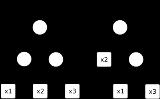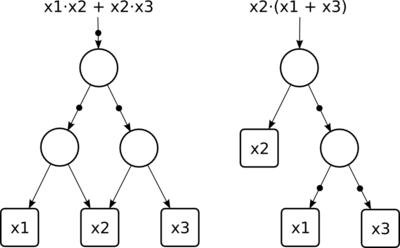xAnd-inverter graphEncyclopedia
An and-inverter graph is a directed, acyclic graph
Graph (mathematics)
In mathematics, a graph is an abstract representation of a set of objects where some pairs of the objects are connected by links. The interconnected objects are represented by mathematical abstractions called vertices, and the links that connect some pairs of vertices are called edges...

that represents a structural implementation of the logical functionality of a circuit or network
Digital circuit
Digital electronics represent signals by discrete bands of analog levels, rather than by a continuous range. All levels within a band represent the same signal state...

. An AIG consists of two-input nodes representing logical conjunction
Logical conjunction
In logic and mathematics, a two-place logical operator and, also known as logical conjunction, results in true if both of its operands are true, otherwise the value of false....

, terminal nodes labeled with variable names, and edges optionally containing markers indicating logical negation. This representation of a logic function is rarely structurally efficient for large circuits, but is an efficient representation for manipulation of boolean functions. Typically, the abstract graph is represented as a data structure
Data structure
In computer science, a data structure is a particular way of storing and organizing data in a computer so that it can be used efficiently.Different kinds of data structures are suited to different kinds of applications, and some are highly specialized to specific tasks...

in software.Conversion from the network of logic gate
Logic gate
A logic gate is an idealized or physical device implementing a Boolean function, that is, it performs a logical operation on one or more logic inputs and produces a single logic output. Depending on the context, the term may refer to an ideal logic gate, one that has for instance zero rise time and...

s to AIGs is fast and scalable. It only requires that every gate be expressed in terms of AND gate
AND gate
The AND gate is a basic digital logic gate that implements logical conjunction - it behaves according to the truth table to the right. A HIGH output results only if both the inputs to the AND gate are HIGH . If neither or only one input to the AND gate is HIGH, a LOW output results...

s and inverters
Inverter (logic gate)
In digital logic, an inverter or NOT gate is a logic gate which implements logical negation. The truth table is shown on the right.This represents perfect switching behavior, which is the defining assumption in Digital electronics. In practice, actual devices have electrical characteristics that...

. This conversion does not lead to unpredictable increase in memory use and runtime. This makes the AIG an efficient representation in comparison with either the binary decision diagram
Binary decision diagram
In the field of computer science, a binary decision diagram or branching program, like a negation normal form or a propositional directed acyclic graph , is a data structure that is used to represent a Boolean function. On a more abstract level, BDDs can be considered as a compressed...

(BDD) or the "sum-of-product" (ΣoΠ) form, that is, the canonical form
Canonical form (Boolean algebra)
In Boolean algebra, any Boolean function can be expressed in a canonical form using the dual concepts of minterms and maxterms. Minterms are called products because they are the logical AND of a set of variables, and maxterms are called sums because they are the logical OR of a set of variables In...

in Boolean algebra known as the disjunctive normal form
Disjunctive normal form
In boolean logic, a disjunctive normal form is a standardization of a logical formula which is a disjunction of conjunctive clauses. As a normal form, it is useful in automated theorem proving. A logical formula is considered to be in DNF if and only if it is a disjunction of one or more...

(DNF). The BDD and DNF may also be viewed as circuits, but they involve formal constraints that deprive them of scalability. For example, ΣoΠs are circuits with at most two levels while BDDs are canonical, that is, they require that input variables be evaluated in the same order on all paths.

Circuits composed of simple gates, including AIGs, are an "ancient" research topic. The interest in AIGs started in the late 1950s and continued in the 1970s when various local transformations have been developed. These transformations were implemented in several
logic synthesis and verification systems, such as Darringer et al. and Smith et al., which reduce circuits to improve area and delay during synthesis, or to speed up formal equivalence checking
Formal equivalence checking
Formal equivalence checking process is a part of electronic design automation , commonly used during the development of digital integrated circuits, to formally prove that two representations of a circuit design exhibit exactly the same behavior....

. Several important techniques were discovered early at IBM
IBM
International Business Machines Corporation or IBM is an American multinational technology and consulting corporation headquartered in Armonk, New York, United States. IBM manufactures and sells computer hardware and software, and it offers infrastructure, hosting and consulting services in areas...

, such as combining and reusing multi-input logic expressions and subexpressions, now known as structural hashing.

Recently there has been a renewed interest in AIGs as a functional representation for a variety of tasks in synthesis and verification. That is because representations popular in the 1990s (such as BDDs) have reached their limits of scalability in many of their applications. Another important development was the recent emergence of much more efficient boolean satisfiability (SAT) solvers. When coupled with AIGs as the circuit representation, they lead to remarkable speedups in solving a wide variety of boolean problems.

AIGs found successful use in diverse EDA
Electronic design automation
Electronic design automation is a category of software tools for designing electronic systems such as printed circuit boards and integrated circuits...

applications. A well-tuned combination of AIGs and boolean satisfiability made an impact on formal verification
Formal verification
In the context of hardware and software systems, formal verification is the act of proving or disproving the correctness of intended algorithms underlying a system with respect to a certain formal specification or property, using formal methods of mathematics .- Usage :Formal verification can be...

, including both model checking
Model checking
In computer science, model checking refers to the following problem:Given a model of a system, test automatically whether this model meets a given specification....

and equivalence checking. Another recent work shows that efficient circuit compression techniques can be developed using AIGs. There is a growing understanding that logic and physical synthesis problems can be solved using AIGs simulation and boolean satisfiability compute functional properties (such as symmetries) and node flexibilities (such as don't-cares, resubstitutions, and SPFDs). This work shows that AIGs are a promising unifying representation, which can bridge logic synthesis
Logic synthesis
In electronics, logic synthesis is a process by which an abstract form of desired circuit behavior, typically register transfer level , is turned into a design implementation in terms of logic gates. Common examples of this process include synthesis of HDLs, including VHDL and Verilog...

, technology mapping, physical synthesis, and formal verification. This is, to a large extent, due to the simple and uniform structure of AIGs, which allow rewriting, simulation, mapping, placement, and verification to share the same data structure.

In addition to combinational logic, AIGs have also been applied to sequential logic
Sequential logic
In digital circuit theory, sequential logic is a type of logic circuit whose output depends not only on the present input but also on the history of the input. This is in contrast to combinational logic, whose output is a function of, and only of, the present input...

and sequential transformations. Specifically, the method of structural hashing was extended to work for AIGs with memory elements (such as D-type flip-flops with an initial state,
which, in general, can be unknown) resulting in a data structure that is specifically tailored for applications related to retiming
Retiming
Retiming is the technique of moving the structural location of latches or registers in a digital circuit to improve its performance, area, and/or power characteristics in such a way that preserves its functional behavior at its outputs. Retiming was first described by Charles E. Leiserson and...

.

Ongoing research includes implementing a modern logic synthesis system completely based on AIGs. The prototype called ABC features an AIG package, several AIG-based synthesis and equivalence-checking techniques, as well as an experimental implementation of sequential synthesis. One such technique combines technology mapping and retiming in a single optimization step. These optimizations can be implemented using networks composed of arbitrary gates, but the use of AIGs makes them more scalable and easier to implement.

## Implementations

• Binary decision diagram
Binary decision diagram
In the field of computer science, a binary decision diagram or branching program, like a negation normal form or a propositional directed acyclic graph , is a data structure that is used to represent a Boolean function. On a more abstract level, BDDs can be considered as a compressed...

• Logical conjunction
Logical conjunction
In logic and mathematics, a two-place logical operator and, also known as logical conjunction, results in true if both of its operands are true, otherwise the value of false....

----